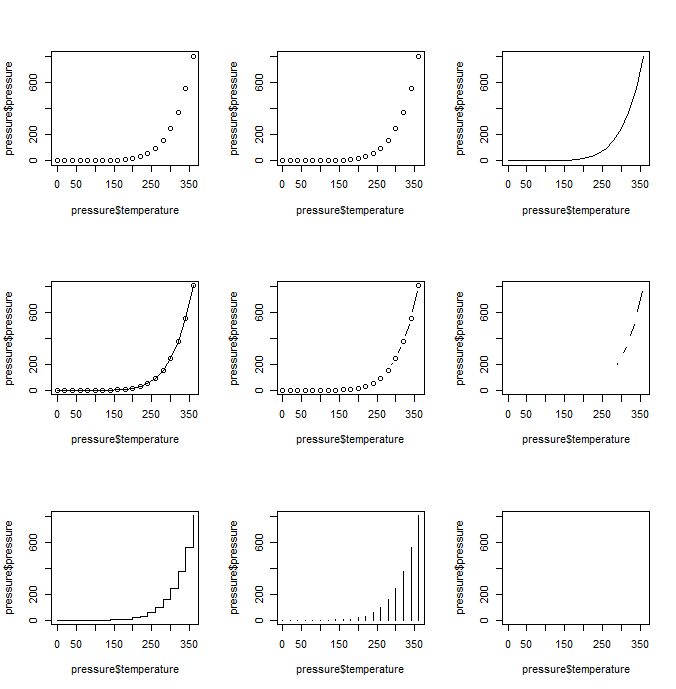# Line Chart

## What is a Line Chart?A line chart is the graphical representation of the functional relationship of two (with 2D representation) or three (with 3D representation) characteristics as a diagram in line form, whereby changes or developments (for example within a certain period of time) can be represented. In contrast to the scatterplot, there can only be one pair or trio of values at a time. If enough data points are collected during a measurement, these points are usually ordered on the x-coordinate and can be connected by drawing line segments between them. Line charts are usually used in identifying the trends in data.

Info
The `lines()` function adds information to a graph. It can not produce a graph on its own.

Usually it follows a `plot(x, y)` command that produces a graph.

By default, `plot( )` plots the (x,y) points. Use the `type="n"` option in the `plot( )` command, to create the graph with axes, titles, etc., but without plotting the points.

`type=` can take the following values:

type description
p points
l lines
o overplotted lines and points
b points joined by lines
c no visible points (empty) joined by lines
s, S line-like stair steps
h histogram-like vertical lines
n does not produce any points or lines

## Example with mtcars:

By default the `plot()` function produces a scatter plot with dots. To make line graphs, pass it to the vector of x and y values, and specify the `type = " "`:

``````plot(x = pressure\$temperature, y = pressure\$pressure)                  # 0.
plot(x = pressure\$temperature, y = pressure\$pressure, type = "p")      # 1.
plot(x = pressure\$temperature, y = pressure\$pressure, type = "l")      # 2.
plot(x = pressure\$temperature, y = pressure\$pressure, type = "o")      # 3.
plot(x = pressure\$temperature, y = pressure\$pressure, type = "b")      # 4.
plot(x = pressure\$temperature, y = pressure\$pressure, type = "c")      # 5.
plot(x = pressure\$temperature, y = pressure\$pressure, type = "s")      # 6.
plot(x = pressure\$temperature, y = pressure\$pressure, type = "h")      # 7.
plot(x = pressure\$temperature, y = pressure\$pressure, type = "n")      # 8.
``````First row from left to right: 0. scatterplot without specification, 1. chart with points (equal to no specification), 2. line chart, 3. overplotted lines and point; second row from left to right: 4. points joined by lines, 5. no visible points (empty) joined by lines, 6. line-like stair steps: third row from left to right: 7. histogram-like vertical points, 8. no visible points or lines since it does not produce any.

To include multiple lines or to plot the points, first call plot() for the first line, then add additional lines and points with lines() and points() respectively:

``````# base graphic
plot(x = pressure\$temperature, y = pressure\$pressure,
type = "l", col = "steelblue")

points(x = pressure\$temperature, y = pressure\$pressure, col = "steelblue")

# add second line in red color
lines(x = pressure\$temperature, y = pressure\$pressure/2, col = "darkgreen")

# add points to second line
points(x = pressure\$temperature, y = pressure\$pressure/2, col = "darkgreen")
``````

## Example with orange:

To demonstrate the creation of a more complex line chart, let’s plot the growth of 5 orange trees over time. Each tree will have its own distinctive line. The data come from the dataset Orange.

``````# convert factor to numeric for convenience
Orange\$Tree <- as.numeric(Orange\$Tree)
ntrees <- max(Orange\$Tree)
``````
``````# get the range for the x and y axis
xrange <- range(Orange\$age)
yrange <- range(Orange\$circumference)
``````
``````# set up the plot
plot(xrange, yrange, type="n", xlab="Age (days)",
ylab="Circumference (mm)" )
colors <- rainbow(ntrees)
linetype <- c(1:ntrees)
plotchar <- seq(18,18+ntrees,1)
``````
``````# add lines via for-loop
for (i in 1:ntrees)
{
tree <- subset(Orange, Tree==i)
lines(tree\$age, tree\$circumference, type="b", lwd=1.5,
lty=linetype[i], col=colors[i], pch=plotchar[i])
}
``````
``````# add a title and subtitle
title("Tree Growth")